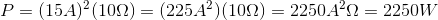# High School Physics : Calculating Circuit Power

## Example Questions

### Example Question #1 : Calculating Circuit Power

What is the power of a circuit with a current of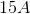and a resistance of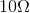?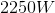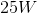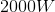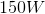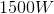Explanation:

The standard equation for power is: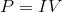We are given only current and reistance, meaning we must manipulate this equation so that only those variables are present. To do this, we can substitute Ohm's law for voltage.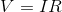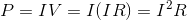Now we have an equation for power that uses only current and resistance. Plug in the values given in the question to find the final answer.